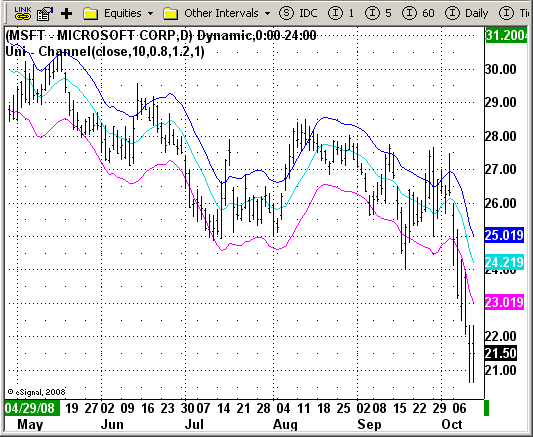# Uni - Channel

ICE Data Services -

 Uni_channel.efs EFSLibrary - Discussion Board

File Name: Uni_channel.efs

Description:
This is the Uni-channel indicator from Ensign. The type designation of 1
means that a constant value is added to the moving average value. Type 2
means that percentage value is added to the moving average.

Formula Parameters:
Moving average source: close
Moving average length: 10
Upper band width: 0.8
Lower band width: 1.2
Mode of indicator: 1

Notes:
Bands define the upper and lower boundaries of a security's normal trading
range. A sell signal is generated when the security reaches the upper band
whereas a buy signal is generated at the lower band. The optimum percentage
shift depends on the volatility of the security--the more volatile, the larger
the percentage.

The logic behind bands is that overzealous buyers and sellers push the price
to the extremes (i.e., the upper and lower bands), at which point the prices
often stabilize by moving to more realistic levels.

There are two kinds of bands: envelopes and Bollinger bands.

The difference between Bollinger bands and envelopes is envelopes are plotted at a
fixed percentage above and below a moving average, whereas Bollinger bands are plotted
at standard deviation levels above and below a moving average. Since standard deviation
is a measure of volatility, the bands are self-adjusting: widening during volatile markets
and contracting during calmer periods. Bollinger Bands were created by John Bollinger.`/*********************************Provided By: eSignal (Copyright c eSignal), a division of Interactive Data Corporation. 2008. All rights reserved. This sample eSignal Formula Script (EFS) is for educational purposes only and may be modified and saved under a new file name. eSignal is not responsible for the functionality once modified. eSignal reserves the right to modify and overwrite this EFS file with each new release.Description: This is the Uni-channel indicator from Ensign. The type designation of 1 means that a constant value is added to the moving average value. Type 2 means that percentage value is added to the moving average.Version: 1.0 09/30/2008Notes: Bands define the upper and lower boundaries of a security's normal trading range. A sell signal is generated when the security reaches the upper band whereas a buy signal is generated at the lower band. The optimum percentage shift depends on the volatility of the security--the more volatile, the larger the percentage. The logic behind bands is that overzealous buyers and sellers push the price to the extremes (i.e., the upper and lower bands), at which point the prices often stabilize by moving to more realistic levels. There are two kinds of bands: envelopes and Bollinger bands. The difference between Bollinger bands and envelopes is envelopes are plotted at a fixed percentage above and below a moving average, whereas Bollinger bands are plotted at standard deviation levels above and below a moving average. Since standard deviation is a measure of volatility, the bands are self-adjusting: widening during volatile markets and contracting during calmer periods. Bollinger Bands were created by John Bollinger.Formula Parameters: Default: Moving average source close Moving average length 10 Upper band width 0.8 Lower band width 1.2 Mode of indicator 1**********************************/var fpArray = new Array();var bInit = false;function preMain() { setPriceStudy(true); setStudyTitle("Uni - Channel"); setCursorLabelName("CentLine", 0); setCursorLabelName("Upper", 1); setCursorLabelName("Lower", 2); setDefaultBarFgColor(Color.aqua, 0); setDefaultBarFgColor(Color.blue, 1); setDefaultBarFgColor(Color.magenta, 2); askForInput(); var x=0; fpArray[x] = new FunctionParameter("Price", FunctionParameter.STRING); with(fpArray[x++]){ setName("Moving average source"); addOption("open"); addOption("high"); addOption("low"); addOption("close"); addOption("hl2"); addOption("hlc3"); addOption("ohlc4"); setDefault("close"); } fpArray[x] = new FunctionParameter("MaLen", FunctionParameter.NUMBER); with(fpArray[x++]){ setName("Average length"); setLowerLimit(1); setDefault(10); } fpArray[x] = new FunctionParameter("Const1", FunctionParameter.NUMBER); with(fpArray[x++]){ setName("Upper band multiplier"); setLowerLimit(0.01); setDefault(0.8); } fpArray[x] = new FunctionParameter("Const2", FunctionParameter.NUMBER); with(fpArray[x++]){ setName("Lower band multiplier"); setLowerLimit(0.01); setDefault(1.2); } fpArray[x] = new FunctionParameter("Type", FunctionParameter.NUMBER); with(fpArray[x++]){ setName("Mode of indicator"); addOption(1); addOption(2); setDefault(1); }}var xPrice = null;var xUpper = null;var xLower = null;var xMA = null;function main(Price, MaLen, Const1, Const2, Type) { var nBarState = getBarState(); if(nBarState == BARSTATE_ALLBARS) { if (Price == null) Price = "close"; if (MaLen == null) MaLen = 10; if (Const1 == null) Const1 = 0.8; if (Const2 == null) Const2 = 1.2; if (Type == null) Type = 1; } if (bInit == false) { xPrice = eval(Price)(); xMA = sma(MaLen, xPrice); if (Type == 2) { xUpper = upperEnv(MaLen, false, Const1, xPrice); xLower = lowerEnv(MaLen, false, Const2, xPrice); } bInit = true; } var nUpper = 0; var nLower = 0; var nMA = xMA.getValue(0); if (Type == 1) { nUpper = nMA + Const1; nLower = nMA - Const2; } else if (Type == 2) { nUpper = xUpper.getValue(0); nLower = xLower.getValue(0); } else return; if (getCurrentBarCount() < MaLen) return; return new Array(nMA, nUpper, nLower);}`